# Notes/calculations specific to each compound

YBa2Cu3O6.92 (Pmmm, TC0meas = 93.78 K)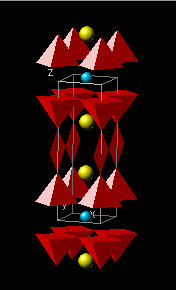To determine σ0, one considers oxygen content x above the minimum value (x0 = 6.35 ) required for superconductivity to occur.  The total oxygen content associated with the optimal superconductive state is then, (6.92 – 6.35) = 0.57.  Given a valence of –2 per oxygen ion, the total number of carriers available to dope the superconducting structure is 2×0.57 = 1.14.  As there are five oxygen-containing layers, two CuO2 layers, two BaO layers and one CuO layer, and assuming that all five layers are populated equally, one then has :

• Type I reservoir: BaO-CuO-BaO
• Type II reservoir: CuO2-Y-CuO2
• σ = σ0 = 1.14/5 = 0.228
• η = 2; ν = 2
• A = 14.8596 Å2; ζ = 2.2677 Å
• TC0calc = 93.36 K
1. D. R. Harshman, A. T. Fiory and J. D. Dow, J. Phys.: Condens. Matt. 23, 295701 (2011)23, 349501 (2011).

YBa2Cu3O6.60 (Pmmm, TC0meas = 63 K)Using rule (2b), σ for the “60 K” phase material can be similarly obtained as with the “90 K” material, but with a participating charge of 2(6.60 – 6.35)/5 = 0.1. However, one obtains the same answer by simply scaling the oxygen (anion) content above x0 = 6.35 relative to that of YBa2Cu3O6.92.  Therefore, one has :

• Type I reservoir: BaO-CuO-BaO
• Type II reservoir: CuO2-Y-CuO2
• σ = [(6.60 – 6.35)/(6.92 – 6.35)] σ0 = 0.439 σ0 = 0.1
• η = 2; ν = 2
• A = 14.8990 Å2; ζ = 2.2324 Å
• TC0calc = 64.77 K
1. D. R. Harshman, A. T. Fiory and J. D. Dow, J. Phys.: Condens. Matt. 23, 295701 (2011)23, 349501 (2011).

LaBa2Cu3O7–δ (Pmmm, TC0meas = 97 K)For this material, the valences and stoichiomentries are unchanged with respect to YBa2Cu3O6.92, such that γ = 1.  Therefore, the optimal transition temperature for this and all other REBa2Cu3O7–δ compounds is completely determined by the structural parameters, A and ζ :

• Type I reservoir: BaO-CuO-BaO
• Type II reservoir: CuO2-Y-CuO2
• σ = σ0
• η = 2; ν = 2
• A = 15.3306 Å2; ζ = 2.1952 Å
• TC0calc = 98.00 K
1. D. R. Harshman, A. T. Fiory and J. D. Dow, J. Phys.: Condens. Matt. 23, 295701 (2011)23, 349501 (2011).

(Ca0.45La0.55)(Ba1.3La0.7)Cu3Oy (Pmmm, TC0meas = 80.5 K)The charge compensated compound, (CaxLa1–x)(Ba1.75–xLa0.25+x)Cu3Oy (or CLBLCO), optimizes for x = 0.45.  To determine γ, one considers only the type I reservoir and its stoichiometric relationship to that of YBa2Cu3O6.92.  From rule (2b) γ is defined by the ratio of the Ba content of CLBLCO (i.e., 1.75 – x) divided by the 2 Ba ions contained in YBa2Cu3O6.92.  Thus, one obtains :

• Type I reservoir: 1/2(Ba1.3La0.7)O-CuO-1/2(Ba1.3La0.7)O
• Type II reservoir: CuO2-(Ca0.45La0.55)-CuO2
• σ = [(1.75 – 0.45)/2] σ0 = 0.65 σ0
• η = 2; ν=2
• A = 15.0118 Å2; ζ = 2.1297 Å
• TC0calc = 82.29 K

Verifying Eq. (2.3) of Ref.  – The equilibrium assertion of equation, νσI = ησII, defines the requirement for achieving an optimal high-TC superconducting state.  It is, therefore, important that its validity be tested.  An ideal material for this exercise is the charge-compensated compound (CaxLa01–x)(Ba1.75–xLa0.25+x)Cu3Oy, for which the doping parameters for La and O are well established . Accepting that the La+3 substituting for Ba+2 and the excess oxygen are associated with the type I reservoir and Ca+2 substituting for La+3 is associated with the type II reservoir, one can write,

νσI =ησII  →  ν(γBa/La) (γO) σ0 = η(γLa/Ca) σ0 ,

where, in this case, ν = η = 2.  The three γ-factors derive from the valence scaling rules as follows:

• γBa/La = (1.75 – x)/2; derived utilizing rule (2b), which scales the outer-layer Ba+2 content [= (1.75 – x)] to that of YBa2Cu3O92 (= 2) .
• γO = [(yy0)/(6.92 – 6.35)]; deduced by taking y = 7.15 ± 0.02 and y0 = 6.88 (at x = 0.40, near optimal stoichiometry) , and scaling to the corresponding oxygen content of YBa2Cu3O6.92 participating in superconductivity .
• γLa/Ca = [1 + (2–3)/3]x = 2x/3; follows from rule (2a) for Ca+2 substituting for La+3 in the type II reservoir. Since both Ca and La have very low and nearly identical electronegativities (1.0 and 1.1, respectively), no additional factors come into play.

By inserting the value for y – y0 (= 0.27) into the above equation, and solving for x, one obtains a value of 0.46 which is in excellent agreement with average value of x = 0.45, thus verifying Eq. (2.3) of Ref. .

1. D. R. Harshman and A. T. Fiory, Phys. Rev. B 86, 144533 (2012).
2. D. R. Harshman, A. T. Fiory and J. D. Dow, J. Phys.: Condens. Matt. 23, 295701 (2011)23, 349501 (2011).

YBa2Cu4O8 (12 GPa) (Ammm, TC0meas = 104 K)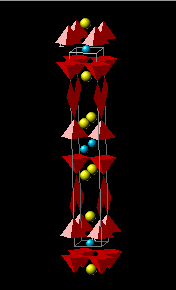For this material, the valences and stoichiomentries are unchanged with respect to YBa2Cu3O6.92, such that γ = 1.  Therefore, the optimal transition temperature for this and all other REBa2Cu3O7–δ compounds is completely determined by the structural parameters, A and ζ :

• Type I reservoir: BaO-CuO-CuO-BaO
• Type II reservoir: CuO2-Y-CuO2
• σ = σ0
• η = 2; ν=2
• A = 14.2060 Å2; ζ = 2.1658 Å
• TC0calc = 103.19 K
1. D. R. Harshman, A. T. Fiory and J. D. Dow, J. Phys.: Condens. Matt. 23, 295701 (2011)23, 349501 (2011).

Tl2Ba2CuO6 (I4/mmm, TC0meas = 80 K)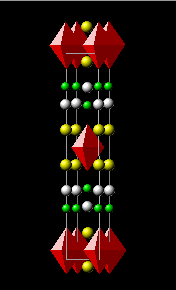Given the +3 charge state of Tl in Tl2Ba2CuO6, compared to Cu+2 in the CuO chain layer of YBa2Cu3O6.92, rule (2a) introduces a factor of 1/2 in γ.  The presence of the double TlO layer structure, in place of the single CuO chain layer in YBa2Cu3O6.92, invokes an additional factor of 2 from rule (2b).  Combining the two factors then yields [1,2]:

• Type I reservoir: BaO-TlO-TlO-BaO
• Type II reservoir: CuO2
• σ = (1/2)(2) σ0 = σ0 
• η = 1; ν=2
• A = 14.9460 Å2; ζ = 1.9291 Å
• TC0calc = 79.86 K
1. D. R. Harshman, A. T. Fiory and J. D. Dow, J. Phys.: Condens. Matt. 23, 295701 (2011)23, 349501 (2011).
2. D. R. Harshman and A. T. Fiory, J. Phys. Chem. Solids 85, 106 (2015).

Tl2Ba2CaCu2O8 (I4/mmm, TC0meas = 110 K)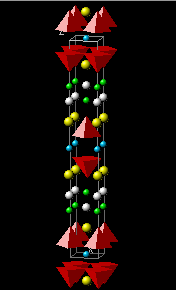Given the +3 charge state of Tl in Tl2Ba2CaCu2O8, compared to Cu+2 in the CuO chain layer of YBa2Cu3O6.92, rule (2a) introduces a factor of 1/2 in γ.  The presence of the double TlO layer structure, in place of the single CuO chain layer in YBa2Cu3O6.92, invokes an additional factor of 2 from rule (2b).  Combining the two factors then yields [1,2]:

• Type I reservoir: BaO-TlO-TlO-BaO
• Type II reservoir: CuO2-Ca-CuO2
• σ = (1/2)(2) σ0 = σ0 
• η = 2; ν=2
• A = 14.8610 Å2; ζ = 2.0139 Å
• TC0calc = 108.50 K
1. D. R. Harshman, A. T. Fiory and J. D. Dow, J. Phys.: Condens. Matt. 23, 295701 (2011)23, 349501 (2011).
2. D. R. Harshman and A. T. Fiory, J. Phys. Chem. Solids 85, 106 (2015).

Tl2Ba2Ca2Cu3O10 (I4/mmm, TC0meas = 130 K)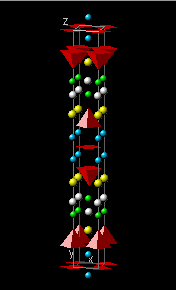Given the +3 charge state of Tl in Tl2Ba2Ca2Cu3O10, compared to Cu+2 in the CuO chain layer of YBa2Cu3O6.92, rule (2a) introduces a factor of 1/2 in γ.  The presence of the double TlO layer structure, in place of the single CuO chain layer in YBa2Cu3O6.92, invokes an additional factor of 2 from rule (2b).  Combining the two factors then yields [1,2]:

• Type I reservoir: BaO-TlO-TlO-BaO
• Type II reservoir:  CuO2-Ca-CuO2-Ca-CuO2
• σ = (1/2)(2) σ0 = σ0 
• η = 3; ν=2
• A = 14.8248 Å2; ζ = 2.0559 Å
• TC0calc = 130.33 K
1. D. R. Harshman, A. T. Fiory and J. D. Dow, J. Phys.: Condens. Matt. 23, 295701 (2011)23, 349501 (2011).
2. D. R. Harshman and A. T. Fiory, J. Phys. Chem. Solids 85, 106 (2015).

TlBa1.2La0.8CuO5 (P4/mmm, TC0meas = 45.4 K)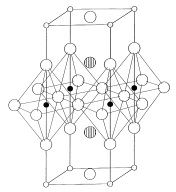Given the +3 charge state of Tl in TlBa1.2La0.8CuO5, compared to Cu+2 in the CuO chain layer of YBa2Cu3O6.92, rule (2a) introduces a factor of 1/2 in γ.  The presence of only 1.2 Ba ions in the outer layers, compare to two in YBa2Cu3O6.92, invokes an additional γ-factor of 1.2/2 = 0.6 from rule (2b).  Combining the two factors then yields [1,2]:

• Type I reservoir: (Ba1.2La0.8)O-TlO-(Ba1.2La0.8)O
• Type II reservoir: CuO2
• σ = (1/2)(0.6) σ0 = 0.300 σ0 
• η = 1; ν=2
• A = 14.7475 Å2; ζ = 1.9038 Å
• TC0calc = 44.62 K
1. D. R. Harshman, A. T. Fiory and J. D. Dow, J. Phys.: Condens. Matt. 23, 295701 (2011)23, 349501 (2011).
2. D. R. Harshman and A. T. Fiory, J. Phys. Chem. Solids 85, 106 (2015).

Tl0.7LaSrCuO5 (P4/mmm, TC0meas = 37 K)Given the +3 charge state of Tl in Tl0.7LaSrCuO5, compared to Cu+2 in the CuO chain layer of YBa2Cu3O6.92, and treating vacancies as non-contributors (i.e., equivalent to a factor of 1/4 – see Bi-2212), rule (2a) introduces a γ-factor of [0.7(1/2) + 0.3(1/4)].  The presence of only 1 Sr+2 ion in the outer layers, compare to two Ba+2 ions in YBa2Cu3O6.92, invokes an additional γ-factor of 1/2 from rule (2b).  Combining the two factors then yields [1,2]:

• Type I reservoir: LaSrO-Tl0.7O-LaSrO
• Type II reservoir: CuO2
• σ = (1/2)[0.7(1/2) + 0.3(1/4)] σ0 = 0.2125 σ0  
• η = 1; ν=2
• A = 14.2453 Å2; ζ = 1.8368 Å
• TC0calc = 39.63 K
1. D. R. Harshman, A. T. Fiory and J. D. Dow, J. Phys.: Condens. Matt. 23, 295701 (2011)23, 349501 (2011).
2. D. R. Harshman and A. T. Fiory, J. Phys. Chem. Solids 85, 106 (2015).

TlBa2CaCu2O7–δ (P4/mmm, TC0meas = 103 K)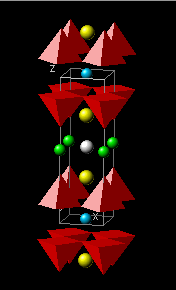In the case of the two Tl-based compounds containing a single TlO layer, TlBa2CaCu2O7–δ (Tl-1212) and TlBa2Ca2Cu3O9–δ (Tl-1223), the mixed-valence nature of Tl places the average Tl oxidation state between f+1 and f+3.  As a consequence, σ becomes a function of the fractional distribution of the monovalent and trivalent Tl oxidation states, which is measured experimentally. Denoting f+1 to be the fraction of monovalent cations in the inner TlO layer (i.e., Tl+1), and apportioning a mixture of +1 and +3 valencies in rule (2a), one has γ = (2)f+1 +  (1/2)(1 – f+1) for these optimal compounds; therefore, σ = γ σ0 = (1.5 f+1 + 0.5) σ0.  Knowing f+1 thus determines σ or, alternatively, f+1 can be deduced for a given value of σ. Given the absence of +3 cations in the outer type I layers, one may assume for argument’s sake that, as with their double-TlO layer counterparts, charge depletion below σ0 does not occur for these compounds; taking optimal σ = σ0 one has [1,2]:

• Type I reservoir: BaO-TlO-BaO
• Type II reservoir: CuO2-Ca-CuO2
• σ = (1.5 f+1 + 0.5) σ0 = σ0 (assumed the same as Tl-1223) 
• f+1 = 1/3; f+3 = 2/3 
• η = 2; ν=2
• A = 14.8734 Å2; ζ = 2.0815 Å
• TC0calc = 104.93 K
1. D. R. Harshman, A. T. Fiory and J. D. Dow, J. Phys.: Condens. Matt. 23, 295701 (2011)23, 349501 (2011).
2. D. R. Harshman and A. T. Fiory, J. Phys. Chem. Solids 85, 106 (2015).

TlBa2Ca2Cu3O9–δ (P4/mmm, TC0meas = 133.5 K)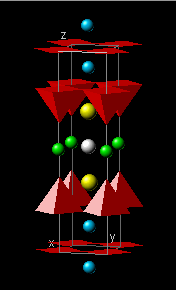In the case of the two Tl-based compounds containing a single TlO layer, TlBa2CaCu2O7–δ (Tl-1212) and TlBa2Ca2Cu3O9–δ (Tl-1223), the mixed-valence nature of Tl places the average Tl oxidation state between f+1 and f+3.  As a consequence, σ becomes a function of the fractional distribution of the monovalent and trivalent Tl oxidation states, which is measured experimentally. Denoting f+1 to be the fraction of monovalent cations in the inner TlO layer (i.e., Tl+1), and apportioning a mixture of +1 and +3 valencies in rule (2a), one has γ = (2)f+1 +  (1/2)(1 – f+1) for these optimal compounds; therefore, σ = γ σ0 = (1.5 f+1 + 0.5) σ0.  Knowing f+1 thus determines σ or, alternatively, f+1 can be deduced for a given value of σ. Given the absence of +3 cations in the outer type I layers, one may assume for argument’s sake that, as with their double-TlO layer counterparts, charge depletion below σ0 does not occur for these compounds; taking optimal σ = σ0 one has [1,2]:

• Type I reservoir: BaO-TlO-BaO
• Type II reservoir: CuO2-Ca-CuO2-Ca-CuO2
• σ = (1.5 f+1 + 0.5) σ0 = σ0 (assumed) 
• f+1 = 1/3; f+3 = 2/3  (shown experimentally) 
• η = 3; ν=2
• A = 14.7686 Å2; ζ = 2.0315 Å
• TC0calc = 132.14 K
1. D. R. Harshman, A. T. Fiory and J. D. Dow, J. Phys.: Condens. Matt. 23, 295701 (2011)23, 349501 (2011).
2. D. R. Harshman and A. T. Fiory, J. Phys. Chem. Solids 85, 106 (2015).

HgBa2Ca2Cu3O8+δ (P4/mmm, δ = 0.27±0.04, TC0meas = 134.43 K)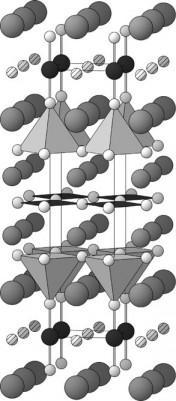For this material, it is assumed that σ = σ0.  Thus one has :

• Type I reservoir: BaO-HgOx-BaO
• Type II reservoir: CuO2-Ca-CuO2-Ca-CuO2
• σ = σ0 (assumed)
• η = 3; ν=2
• A = 14.8060 Å2; ζ = 1.9959 Å
• TC0calc = 134.33 K
1. D. R. Harshman, A. T. Fiory and J. D. Dow, J. Phys.: Condens. Matt. 23, 295701 (2011)23 349501 (2011).

HgBa2Ca2Cu3O8+δ (25 GPa) (P4/mmm, δ = 0.27±0.04, TC0meas = 145 K)For this material, it is assumed that σ = σ0, where the increase in TC0calc over the 0 GPa value is directly related to the decrease in A and ζ . Thus one has :

• Type I reservoir: BaO-HgOx-BaO
• Type II reservoir: CuO2-Ca-CuO2-Ca-CuO2
• σ = σ0 (assumed)
• η = 3; ν=2
• A = 13.6449 Å2; ζ = 1.9326 Å
• TC0calc = 144.51 K
1. D. R. Harshman, A. T. Fiory and J. D. Dow, J. Phys.: Condens. Matt. 23, 295701 (2011)23 349501 (2011).

HgBa2CuO4.15 (P4/mmm, TC0meas = 95 K)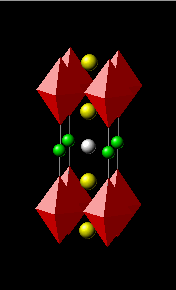We know of at least two optimal compounds for which σ > σ0, HgBa2CuO4.15 and HgBa2CaCu2O6.22, TC0 = 95 K and 127 K, respectively, where the two electrons per excess oxygen (0.15 and 0.22, respectively) are equally distributed among the (3+η) layers (this is equivalent to dividing the carriers between the two reservoirs, and then by the average number of layers per reservoir).  For HgBa2CuO4.15, one then finds that σ = σ0 + 2(0.15)/(3+1) = 0.3030.  This enhancement in TC0meas is attributed to the unique structure of the Hg compounds which provides vacancy locations for excess oxygen and the ≤ 2 valence of Hg. Thus one has :

• Type I reservoir: BaO-HgO-BaO
• Type II reservoir: CuO2
• σ = σ = σ0 + 2(0.15)/(3+1) = σ0 + 0.075 = 0.3030
• η = 1; ν=2
• A = 15.0362 Å2; ζ = 1.9214 Å
• TC0calc = 92.16 K
1. D. R. Harshman, A. T. Fiory and J. D. Dow, J. Phys.: Condens. Matt. 23, 295701 (2011)23, 349501 (2011).

HgBa2CaCu2O6.22 (P4/mmm, TC0meas = 127 K)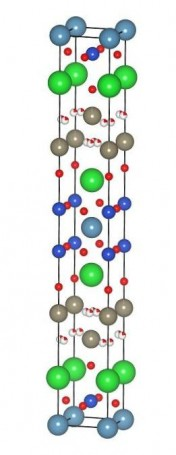We know of at least two optimal compounds for which σ > σ0, HgBa2CuO4.15 and HgBa2CaCu2O6.22, TC0 = 95 K and 127 K, where the two electrons per excess oxygen (0.15 and 0.22, respectively) are equally distributed among the (3+η) layers (this is equivalent to dividing the carriers between the two reservoirs, and then by the average number of layers per reservoir).  For HgBa2CaCu2O6.22, one then finds that σ = σ0 + 2(0.22)/(3+1) = 0.3160.  This enhancement in TC0meas is attributed to the unique structure of the Hg compounds which provides vacancy locations for excess oxygen and the ≤ 2 valence of Hg. Thus one has :

• Type I reservoir: BaO-HgO-BaO
• Type II reservoir: CuO2-Ca-CuO2
• σ = and σ = σ0 + 2(0.22)/(3+2) = σ0 + 0.088 = 0.3160,
• η = 2; ν=2
• A = 14.9375 Å2; ζ = 2.0390 Å
• TC0calc = 125.84 K
1. D. R. Harshman, A. T. Fiory and J. D. Dow, J. Phys.: Condens. Matt. 23, 295701 (2011)23, 349501 (2011).

La1.837Sr0.163CuO4–δ (I4/mmm, TC0meas = 38 K)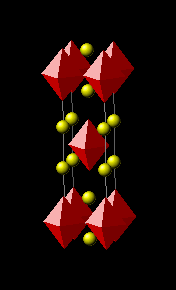Since x0 = 0, the total charge doping is 0.163.  This charge is accordingly distribution between the type I and type II reservoirs [rule (1b)], thereby introducing a factor of 1/2 in γ [rule (1b)].  As there are two SrO layers in the type I reservoir (i.e., ν = 2) rule (1a) provides an additional factor of 1/2 such that :

• Type I reservoir: (La1.837Sr0.163)O-(La1.837Sr0.163)O
• Type II reservoir: CuO2
• σ = (0.163)(1/2)(1/2) = 0.048
• η = 1; ν = 2
• A = 14.2268 Å2; ζ = 1.7828 Å
• TC0calc = 37.47 K
1. D. R. Harshman, A. T. Fiory and J. D. Dow, J. Phys.: Condens. Matt. 23, 295701 (2011)23 349501 (2011).

La1.8Sr0.2CaCu2O6±δ (I4/mmm, TC0meas = 58 K)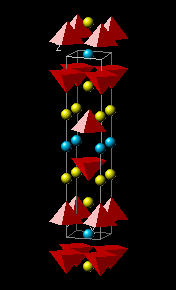Since x0 = 0, the total charge doping is 0.2.  This charge is accordingly distribution between the type I and type II reservoirs, thereby introducing a factor of 1/2 in γ [rule (1b)].  As there are two SrO layers in the type I reservoir (i.e., ν = 2) rule (1a) provides an additional factor of 1/2 such that :

• Type I reservoir: (La1.8Sr0.2)O-(La1.8Sr0.2)O
• Type II reservoir: CuO2-Ca-CuO2
• σ = (0.2)(1/2)(1/2) = 0.05
• η = 2 ; ν = 2
• A = 14.3761 Å2; ζ = 1.7829 Å
• TC0calc = 58.35 K
1. D. R. Harshman, A. T. Fiory and J. D. Dow, J. Phys.: Condens. Matt. 23, 295701 (2011)23, 349501 (2011).

(Sr0.9La0.1)CuO2 (P4/mmm, TC0meas = 43 K)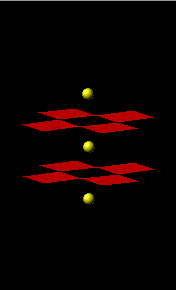For this material, superconductivity occurs with La doping (with x0 = 0).  Given that the total charge doping is 0.1, which is distributed equally between the type I and type II reservoir, rule (1b) introduces a factor of 1/2 in γ.  Since ν = 1, there are no additional γ-factors such that :

• Type I reservoir: (Sr0.9La0.1)
• Type II reservoir: CuO2
• σ = (0.1)(1/2) = 0.05
• η = 1; ν = 1
• A = 15.6058 Å2; ζ = 1.7051 Å
• TC0calc = 41.41 K
1. D. R. Harshman, A. T. Fiory and J. D. Dow, J. Phys.: Condens. Matt. 23, 295701 (2011)23 349501 (2011).

Ba2Y(Ru0.9Cu0.1)O6 (Fm3m, TC0meas = 35 K)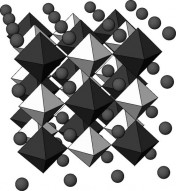The ruthenate compounds A2Y(Ru0.9Cu0.1)O6 (with A = Ba or Sr; x = 0.05–0.15) are double-perovskites containing no cuprate planes and with η = ν = 1 (reference  in original paper ).  The determination of γ is done using charge allocation, wherein rule (1b) introduces the factor 1/2.  In the lower limit, one expects a minimum of ∼ 2 charges per Cu dopant, which are shared between two charge reservoirs of each layer type [AO and 1/2 (YRu1–xCuxO4)], producing a net factor of unity.  Thus, for Ba2YRu0.9Cu0.1O6 (with TC0meas ∼ 30–40 K), we have γ = (1/2)(1) = 1/2, as stated by equation in the original paper of .  The corrigendum also corrects a typographical correction in the definition of the corresponding ruthenate type II reservoir in the last column of table 1 in the original paper , which should read 1/2 (YRu0.9Cu0.1O4).  Thus one has :

• Type I reservoir: BaO
• Type II reservoir: ½(YRu0.9Cu0.1O4)
• σ = (0.1)(1/2) = 0.05
• η = 1; ν = 1
• A = 17.3208 Å2; ζ = 2.0809 Å
• TC0calc = 32.21 K
1. D. R. Harshman, A. T. Fiory and J. D. Dow, J. Phys.: Condens. Matt. 23, 295701 (2011)23, 349501 (2011).

(Pb0.5Cu0.5)Sr2(Y/Ca)Cu2O7–δ (P4/mmm; TC0meas = 67 K)As the description reported in the original work  was a bit misleading, so a correct discussion is given here .  The (Pb0.5Cu0.5.)Sr2 structure makes up the type I reservoir in this material, with both Pb and Cu possessing a +3 charge state (primarily, e.g., from Pb-O bond length measurements). Using Stoichiometric (valency) scaling with respect to YBa2Cu3O6.92, rule (2a) introduces a factor of 1/2 for both.  However, the large electronegativity (see discussion of Bi-2212 in Ref. ) for Pb (χ = 2.33) when compared with Cu (χ = 1.90), an additional factor of 1/2 must accompany the Pb component, such that, γ = (1/2)(0.5Pb/2 + 0.5Cu) = (1/2)(3/4).  Thus one has [1-3]:

• Type I reservoir: SrO-(Pb0.5Cu0.5)O-SrO
• Type II reservoir: CuO2-(Y/Ca)-CuO2
• σ = (1/2)(3/4) σ0 = 0.375 σ0
• η = 2; ν=2
• A = 14.5771 Å2; ζ = 1.9967 Å
• TC0calc = 67.76 K
1. D. R. Harshman, A. T. Fiory and J. D. Dow, J. Phys.: Condens. Matt. 23, 295701 (2011)23, 349501 (2011).
2. D. R. Harshman and A. T. Fiory, J. Phys. Chem. Solids 85, 106 (2015).
3. Unpublished (2014).

Bi2Sr2CaCu2O8+δ (N Bbmb/111, TC0meas = 89 K)In Bi2Sr2(Ca,Y)xCu2O8+δ (Bi-2212), where excess oxygen located in the Bi2O2 block, as well as cation stoichiometry and substitutional defects, are correlated with a strong incommensurate modulation along the b-axis of the basal plane [1,2].  This extra oxygen content, which appears to be enhanced (along with TC) by increasing the Ca vacancies (for x < 1, with zero Y content) or the Y/Ca substitution ratio (x ≈ 1), introduces additional carriers for which the cation scaling rules alone do not account (accurate knowledge of the excess oxygen content in the Bi2O2 block would be required in order to apply anion scaling).  Variation of ζ for non-stoichiometric compositions would also come into play. Consequently, cation scaling to YBa2Cu3O6.92 is applicable only the near stoichiometric Bi2Sr2CaCu2O8+δ, compound (as-grown and unannealed), assuming minimal extra-oxygen enhancement in carrier density, and having a measured transition of 89 K .  Given that there are two BiO layers compared to the one CuO chain in YBa2Cu3O6.92, rule (2b) introduces a γ-factor of 2, and since Bi+3 is mapped onto the chain Cu+2 cation, rule (2a) provides another factor of 1/2.  Finally, because the electronegativity  of Bi (χ = 2.02) is significantly greater than that of Cu (χ = 1.90), charge transfer along the hard axis is suppressed, generating another factor of 1/2.  Utilizing more recent structural refinement data specific to a near-stoichiometric single-crystal sample at 12 K (see Ref. ), one then has [1,2]:

• Type I reservoir: SrO-BiO-BiO-SrO
• Type II reservoir: CuO2-Ca-CuO2
• σ = (2)(1/2)(1/2) σ0 = 0.5 σ0
• η = 2; ν=2
• A = 14.5201 Å2; ζ = 1.7500 Å 
• TC0calc = 89.32 K 

Note that the value of 86.65 K originally published in Ref.  was obtained using structural data for a nonstoichiometric powder sample.

1. D. R. Harshman, A. T. Fiory and J. D. Dow, J. Phys.: Condens. Matt. 23, 295701 (2011)23, 349501 (2011).
2. D. R. Harshman and A. T. Fiory, J. Phys. Chem. Solids 85, 106 (2015).

(Bi/Pb)2Sr2Ca2Cu3O10+δ (Fmmm, TC0meas = 112 K)Given that there are two BiO layers compared to the one CuO chain in YBa2Cu3O6.92, rule (2b) introduces a γ-factor of 2, and since Bi+3 is mapped onto the chain Cu+2 cation, rule (2a) provides another factor of 1/2.  Finally, because the electronegativity (see discussion of Bi-2212 in Ref. ) of Bi (χ = 2.02) is significantly greater than that of Cu (χ = 1.90), charge transfer along the hard axis is suppressed, generating another factor of 1/2.  Thus, one has [1,2]:

• Type I reservoir: SrO-(Bi/Pb)-(Bi/Pb)-SrO
• Type II reservoir: CuO2-Ca-CuO2-Ca-CuO2
• σ = (2)(1/2)(1/2) σ0 = 0.5 σ0
• η = 3; ν=2
• A = 14.6340 Å2; ζ = 1.6872 Å
• TC0calc = 113.02 K
1. D. R. Harshman, A. T. Fiory and J. D. Dow, J. Phys.: Condens. Matt. 23, 295701 (2011)23, 349501 (2011).
2. D. R. Harshman and A. T. Fiory, J. Phys. Chem. Solids 85, 106 (2015).

Pb2Sr2(Y/Ca)Cu3O8 (TC0meas = 75 K)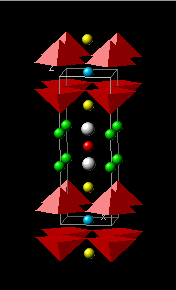Given that there are two PbO layers compared to the one CuO chain in YBa2Cu3O6.92, rule (2b) introduces a γ-factor of 2.  Assuming a +3 average charge state for Pb, and since Pb+3O is mapped onto the chain Cu+2O, rule 2(a) provides a factor of 1/2.  Finally, because the relative electronegativity (see discussion of Bi-2212 in Ref. ) of Pb (χ = 2.33) is significantly greater than that of Cu (χ = 1.90), charge transfer along the hard axis is suppressed, generating another factor of 1/2.  Thus, one has [1,2]:

• Type I reservoir: SrO-PbO-Cu-PbO-SrO
• Type II reservoir: CuO2-(Y/Ca)-CuO2
• σ = (2)(1/2)(1/2) σ0 = 0.5 σ0
• η = 2; ν=2
• A = 14.6458 Å2; ζ = 2.0280 Å
• TC0calc = 76.74 K
1. D. R. Harshman, A. T. Fiory and J. D. Dow, J. Phys.: Condens. Matt. 23, 295701 (2011)23, 349501 (2011).
2. D. R. Harshman and A. T. Fiory, J. Phys. Chem. Solids 85, 106 (2015).

Bi2(Sr1.6La0.4)CuO6+δ (Cmmm, TC0meas = 34 K)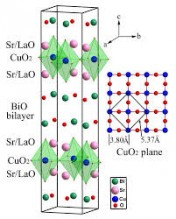For the single-layer material, Bi2(Sr1.6La0.4)CuO6+δ, the 1/2 γ-factor (relative to YBa2Cu3O6.92) in rule (2a) arising from the presence of a double BiO layer structure (in place of a single CuO chain layer), would naturally apply.  However, since YBa2Cu3O6.92 has two corresponding Ba+2 ions, the partial Sr+2 doping (x = 1.6) of the outer layers introduces a relative doping factor of ( 1.6 − 1.16 ) = 0.44 according to rule (2b), where x0 = 1.16.  Finally, the comparatively large electronegativity (see discussion of Bi-2212 in Ref. ) of Bi (χ = 2.02) to that of Cu (χ = 1.90) yields [1,2]:

• Type I reservoir: ½(Sr1.6La0.4)-BiO-BiO-½(Sr1.6La0.4)
• Type II reservoir: CuO2
• σ = (1/2)(0.44)(1/2) σ0 = 0.11 σ0
• η = 1; ν=2
• A = 14.5422 Å2; ζ = 1.4880 Å
• TC0calc = 34.81 K
1. D. R. Harshman, A. T. Fiory and J. D. Dow, J. Phys.: Condens. Matt. 23, 295701 (2011)23, 349501 (2011).
2. D. R. Harshman and A. T. Fiory, J. Phys. Chem. Solids 85, 106 (2015).

RuSr2GdCu2O8 (P4/mmm, TC0meas = 50 K)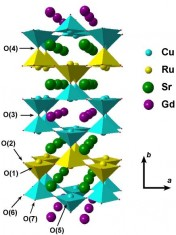In the case of the ruthenocuprate compound, RuSr2GdCu2O8, the structure contains a type I reservoir SrO–RuO2–SrO, where the Cu+2O chain layer is replaced by a Ru+5O2 layer, and Y+3 is replaced by Gd+3. Taking the Ru charge state to be +5 in this material, and given the charge equivalence of Bi+3O−2 and Ru+5(O–2)2, one can draw an analogy with the Bi/Pb compounds, and approximate σ.  In this case, however, there is only one layer that is charge equivalent.  Thus, from rule (2a), one factor of 1/2 is introduced arising from the valency scaling between Ru+5(O–2)2 and Cu+2O,  Given that the Pauling electronegativity (see discussion of Bi-2212 in Ref. ) of Ru is 2.20, which is significantly greater than that of Cu (χ = 1.90), charge transfer aling the hard axis is suppressed, invoking an additional factor of 1/2, such that [1,2]

• Type I reservoir: SrO-RuO2-SrO
• Type II reservoir: CuO2-Gd-CuO2
• σ = (1/2)(1/2) σ0 = 0.25 σ0
• η = 2; ν=2
• A = 14.7372 Å2; ζ = 2.1820 Å
• TC0calc = 50.28 K
1. D. R. Harshman, A. T. Fiory and J. D. Dow, J. Phys.: Condens. Matt. 23, 295701 (2011)23, 349501 (2011).
2. D. R. Harshman and A. T. Fiory, J. Phys. Chem. Solids 85, 106 (2015).

(Ln/Th)(Ox–yF1–x)FeAs (P4/nmm, TC0meas = 26 K – 55 K)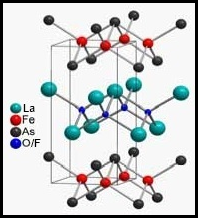In the (n-type) Ln–O/F–Fe–As (Ln-1111) iron pnictides, e.g., La(O0.92−yF0.08)FeAs (TC0meas = 26 K), Ce(O0.84−yF0.16)FeAs (TC0meas = 35 K), Tb(O0.80−yF0.20)FeAs (TC0meas = 45 K) and Sm(O0.65 −yF0.35)FeAs (TC0meas = 55 K), where y accounts for the actual O-site occupancy; we assume [x − x0] is given by F stoichiometry, the Coulombic interaction is assumed to occur between the Ln(O/F) and AsFe layers, which defines ζ (Ln–As distance along the c-axis), and sets η = ν = 1 (i.e. within the periodicity d). Applying rules (1b) (equally shared doping between the hole and electron reservoirs) and (1a) (where the doping is further divided between component layers of the two reservoirs, [(O/F)Ln and (AsFe)], one obtains γ = (1/2)(1/2) = (1/4).  For Ln = Nd, see Ref. .  For the Th+4 doped n-type 1111 iron pnictide compounds, such as (Sm0.7Th0.3)OFeAs (TC0meas = 51.5 K), the value of σ is given similarly, but with [x − x0] determined by the Th doping content.  Thus one has [1, 2]: [Need to recheck structure parameters]

• Type I reservoir: ½(Ln-2O/F-Ln)
• Type II reservoir: ½(As-2Fe-As)
• σ = (1/2)(1/2) [x – x0]; for O-deficient (no F), σ = (1/2)(1/2) (2) [x – x0]
• η = 1; ν = 1
• NbFeAsO0.85-y: A = 15.5417 Å2; ζ = 1.653 Å; TC0calc = 52.42 K 
• GdFeAsO0.85-y: A = 15.1399 Å2; ζ = 1.616 Å; TC0calc = 54.33 K 
• La(O0.92−yF0.08)FeAs: A = 16.1620 Å2; ζ = 1.7677 Å; TC0calc = 24.82 K
• Ce(O0.84−yF0.16)FeAs: A = 15.8778 Å2; ζ = 1.6819 Å; TC0calc = 37.23 K
• Tb(O0.80−yF0.20)FeAs: A = 14.8996 Å2; ζ = 1.5822 Å; TC0calc = 45.67 K
• Nb(O0.70-yF0.30)FeAs: A = 15.626 Å2; ζ = 1.653 Å; TC0calc = 52.28 K 
• Sm(O0.65−yF0.35)FeAs: A = 15.4535 Å2; ζ = 1.6670 Å; TC0calc = 56.31 K
• (Sm0.7Th0.3)OFeAs: A = 15.4897 Å2; ζ = 1.6710 Å; TC0calc = 51.94 K
1. D. R. Harshman, A. T. Fiory and J. D. Dow, J. Phys.: Condens. Matt. 23, 295701 (2011)23, 349501 (2011).
2. D. R. Harshman and A. T. Fiory. preprint (2021).

(Ba/K)(Fe/Co)2As2 (I4/mmm, TC0meas = 37 K & 22 K)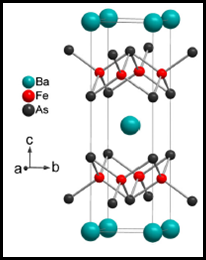As in the case of the 1111 structures, rules (1a) and (1b) also apply in determining γ for the 122 compounds, e.g., (Ba0.6K0.4)Fe2As2 and Ba(Fe1.84Co0.16)As2, except that the doping is also shared between the two FeAs structures [rule (1a)] yielding an additional factor of 1/2 such that γ = (1/2)(1/2)(1/2) = 0.125.  For (Ba0.6K0.4)Fe2As2 (TC0meas = 37 K, x = 0.4 and x0 = 0), the Coulombic interaction occurs between the Ba/K layer and the two adjacent Fe+2As–3 structures, where we designate the Ba(K) layers as type II ( η = 1), which interact with the As in the FeAs type I (ν = 2) structures. The γ factor for the n-type homologue to the above p-type compound, Ba(Fe1.84Co0.16)As2 (TC0meas = 22 K, x = 0.16 and x0 = 0), is similarly calculated, but with [x − x0] corresponding to Co doping. Therefore :

• Type I reservoir: As-2(Fe/Co)-As
• Type II reservoir: Ba/K
• σ = (1/2)(1/2)(1/2) [x − x0]
• η = 1; ν = 2
• (Ba0.6K0.4)Fe2As2: A = 15.2803 Å2; ζ = 1.932 Å; TC0calc = 36.93 K
• Ba(Fe1.84Co0.16)As2: A = 15.6848 Å2; ζ = 1.8920 Å; TC0calc = 23.54 K
1. D. R. Harshman, A. T. Fiory and J. D. Dow, J. Phys.: Condens. Matt. 23, 295701 (2011)23, 349501 (2011).

FeSe0.977 (7.5 GPa) (Pnnm, TC0meas = 36.5 K) [Check structure information]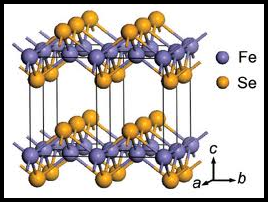While superconducting (tetragonal) Fe1+xSe1−y is very nearly stoichiometric, some degree of symmetry breaking, arising from either a Se deficiency or an excess in Fe content, must exist to induce the superconducting condensate. It is also clear that the optimal superconducting state is achieved at a hydrostatically applied pressure of 7.5 – 8.5 GPa, which we attribute to pressure-induced charge redistribution, analogous to the response of YBa2Cu4O8. Although assigning reservoir types to the Fe and Se layers is somewhat subjective, it is also not a necessary requirement in determining σ (for convention, we refer to the negative valence Se layer as type I). Given the +2 and −2 valences of Fe and Se, respectively, the charge doping is 2x or 2y (or, typically, the sum thereof), where x and y are small and positive quantities. High resistivity (∼33 mΩcm at T ∼ 9 K) observed confirms that stoichiometric FeSe is dominantly a non-metallic material. One may therefore assume that the FeSe binary compound of precisely 1:1 stoichiometry is effectively an insulating material and that doping is achieved by introducing excess Fe or depleted Se. From rule (1b) the dopant charge populates both charge reservoirs, yielding γ = 1/2.  For FeSe0.977 (y = 0.023, y0 = 0), the doping factor has the single term [2(y−y0)] = 2(0.023) and the fractional charge is then calculated to be σ = 2(0.023)/2 = 0.023.  The basal-plane lattice parameter at 7.5 GPa is a = 3.622 A.  The interaction distance ζ is measured between nearest-neighbor Fe and Se ions observed at the same pressure.  Thus one has :

• Type I reservoir: Se0.977
• Type II reservoir: Fe1.0
• σ = (1/2) [2(y − y0)] = 0.023
• η = 1; ν = 1
• A = 13.1189 Å2; ζ = 1.4240 Å
• TC0calc = 36.68 K
1. D. R. Harshman and A. T. Fiory, J. Phys.: Condens. Matt. 24, 135701 (2012).

Fe1.03Se0.57Te0.43 (2.3 GPa) (TC0meas = 23.3 K) [Check structure information]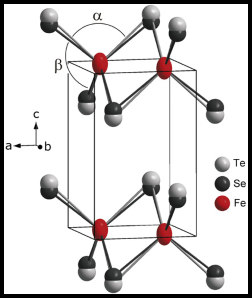To calculate σ for Fe1+xSe1–yTey system (for y > 0.3), one must consider the type I reservoir structure to be Tey–Fex–Se1−y, where ν = 2 and the charge doping, supplied by the excess Fe+2 ions (two charges per excess Fe cation), is shared equally between the Tey and Se1−y layers, with comparable occupancies since y ∼ 1/2.  The result for γ is a factor of 1/2 from rule (1a), multiplied by an additional factor of 1/2 associated with rule (1b), yielding γ = 1/4. Since accurate information on Fe stoichiometry is essential, we consider results reported for a sample with composition Fe1.03Se0.57Te0.43, where x ≈ 0.03 was obtained from pressure- and temperature-dependent Rietveld refinements of synchrotron x-ray powder diffraction data. The sample under study exhibited superconducting transitions (determined from magnetization onsets) of 13.9 K at ambient pressure, reaching a maximum TC = 23.3 K under hydrostatic pressure of 2.3 GPa. Unfortunately, only resistance and zero-field-cooled magnetization data are given, limiting our ability to quantify the sample’s quality.  In another work on an FeSe0.5Te0.5 sample (in this case Fe stoichiometry of unity was apparently presumed, leaving σ undetermined), the superconducting transitions (onset values) varyd from 13.5 K at ambient pressure, reaching a maximum TC of ~25–26 K (26.2 K reported) at 2 GPa.  The sample in this case was judged by the authors to be of high quality, based on a 12% Meissner fraction (the field-cooled magnetization is about 38% of the zero-field-cooled magnetization; normal-state resistivity is 1.4 mΩcm extrapolated to TC at 2 GPa).  Since one does not expect the structural parameters to differ greatly between these two samples, it would normally be reasonable to accept 25.5 K as representative of the highest TC attained for this compound.  Given that the Fe content is unknown for the latter, results from the former are adopted for doping and TC0meas, with the understanding that the quoted transition temperature may be lower than optimum.  To calculate TC0 for the Fe1.03Se0.57Te0.43 compound, we use data taken at 2.0 GPa; a = 3.7317 and b = 3.7262 Å, giving a plane area (per formula unit) of A = 13.9051 Å2.  One further estimates an average interaction distance 〈ζ〉 = 1.597 Å (assuming the measurement reflects an average between ζSe and ζTe).  This value is corroborated elsewhere, which indicates ζTe = 1.7638 Å and ζSe = 1.4162 Å for a sample indicates ζTe = 1.7638 A under pressure (presumably 2.0 GPa), corresponding to an average value 〈ζ〉 = 1.59 Å. Using 〈ζ〉 takes into account disorder in site occupancy and interactions contributed by the further Te ion, given that (ζTe − ζSe) << 〈ζ〉.  Above ∼2.5 GPa, the compound undergoes an orthorhombic to monoclinic transition, resulting in small abrupt changes to some of the relevant lattice parameters.

• Type I reservoir: Se0.57-Fe0.03-Te0.43
• Type II reservoir: Fe1.0
• σ = (1/2) (1/2) [2(1.03 – 1)] = 0.015
• η = 1; ν = 2
• A = 13.9051 Å2; 〈ζ〉 = 1.5970 Å
• TC0calc = 25.65 K
1. D. R. Harshman and A. T. Fiory, J. Phys.: Condens. Matt. 24, 135701 (2012).

AzFe2-xSe2 (TC0meas = 28.5 – 31.5 K)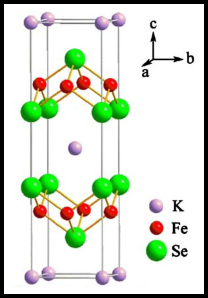Unlike Fe1+xSe1−y, the ternary AzFe2−xSe2 series of compounds can be optimized at ambient pressure, and are characterized by a rather large normal-state resistivity at TC, signifying a higher scattering rate. For these materials, the type I reservoir structures are identified as the Se–Fe2−x–Se triple layers, with ν = 2, and the alkali metal Az layers are defined as the type II (η = 1) reservoir structures (the exact formula-unit structure that we consider is Fe1−x/2–Se–Az–Se–Fe1−x/2, or equivalently Az/2–Se–Fe2−x–Se–Az/2 , the latter corresponding to figure 1(b) in Ref. ). There are generally two doping sources; one associated with the type I reservoir and the other with the type II. The two terms in equation (2) are [z0 − z] and +2[x0 − x] for Az and Fe2−x, respectively, where the prefactor of 2 corresponds to the double valence of Fe (the terms vi (x − x0)i for the two reservoir types have opposite signs). There also appear to be (at least) two insulating end materials corresponding to the alkali metal and iron components (determined for A = K): KFe2Se2 such that z0 = 1, and KFe2−x0Se2, where 0.40 ≤ x0 ≤ 0.42. Since x and z depend on growth stoichiometries, these two doping sources generally provide unequal contributions to the fractional charge, which is found by combining the magnitudes of the two charges as per equation (2), and treating doping as if from a single source. Thus, by analogy with (Ba1−xKx)Fe2As2, the charge allocation rules (1a) and (1b) apply. Here, one factor of 1/2 arises from rule (1b) requiring that the doping charge be shared between the two reservoirs; the factor of (1/2)(1/2) arises from dividing the charge among the four individual layers of the two Fe–Se structures of the type I reservoir (rule (1a) for N = 4, or applied twice with N = 2). From this we can write γ = (1/2)(1/2)(1/2) = 0.125. Thus one has :

• Type I reservoir: Se-Fe2-x-Se
• Type II reservoir: Az
• σ = (1/2) (1/2) (1/2) [(1 – z) + 2(0.40 – x)]
• η = 1; ν = 2
• K0.83Fe1.66Se2: A = 15.2432 Å2; 〈ζ〉 = 2.0241 Å; TC0meas = 29.5 K; TC0calc = 30.07 K
• Rb0.83Fe1.70Se2: A = 15.4867 Å2; 〈ζ〉 = 2.1463 Å; TC0meas = 31.5 K; TC0calc = 31.78 K
• Cs0.83Fe1.71Se2: A = 16.1419 Å2; 〈ζ〉 = 2.3298 Å; TC0meas = 28.5 K; TC0calc = 29.44 K
1. D. R. Harshman and A. T. Fiory, J. Phys.: Condens. Matt. 24, 135701 (2012).

Na0.16(S)yTiNCl (S = PC, BC ) (TC0meas ~ 6 – 8 K)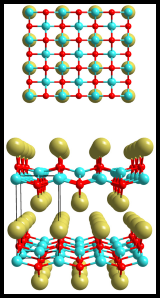High-TC superconductivity in this model occurs in layered structures forming adjacent type-I and type-II charge reservoir layers containing the superconducting and mediating charges, respectively, repeating alternately along the transverse axis. The superconducting transition temperature depends on the spatially indirect Coulomb interaction across the transverse distance ζ between the two charge reservoirs, measured between the outer chlorines in the type-I [TiNCl]2 layer and the locus of the cations Ax in the neighboring type-II intercalation layer, assuming co-intercalant (S)y is uncharged. The layered structure of Ax(S)yTiNCl is characterized by a thickness d2 of the [TiNCl]2 layers, the transverse spacing d between them, and an intercalant thickness dd2. Assuming that the mean cation Ax locus is at the intercalant-layer midplane, the interaction distance is ζ = (dd2)/2. Since d2 is approximately the same as for pristine α-TiNCl (see  of Ref. ), the observed functional dependence of TC on d is expected to correlate with an analogous dependence on ζ . However, one notes that the interlayer interaction length is the shorter distance ζ, rather than the spacing d. Structural and superconductivity data are presented in Table I, listing directly measured values of d2 where available. The Coulomb energy e2/ζ lies within 1.8 – 8.7 eV. For Ax(S)yTiNCl, doping occurs only in the intercalation layer via Ax such that σ is determined according to the simplified relation, σ = γ |v(x − x0)|, where v is the valence and x is the optimal content of the cation dopant species in the type II Ax(S)y reservoir; x0 is the threshold value of x for superconductivity; here, v = 1 for alkali-ion doping and x0 = 0 is inferred elsewhere. The factor γ derives from the allocation of the dopant by considering a given compound’s structure. Following the procedure generally applied to high-TC superconductors, the charge introduced by the dopant is shared equally between the two charge reservoirs [rule (1b)]. Additionally, the methodology requires the doped charge to be distributed pairwise between the charge-carrying layer types within each of the charge reservoirs. Assuming the co-intercalant contributes no doping charge, determination of γ for Ax(S)yTiNCl is comparable to that of (Ba0.6K0.4)Fe2As2 for which a structural analogy was previously noted. Sharing the charge equally between the two reservoirs contributes a factor of 1/2 to γ . Sharing between the Cl layer and the double-TiN-layered structure and then to the two TiN layers contributes two factors from rule (1a) of 1/2 to γ. Hence, γ = (1/2)(1/2)(1/2) = 1/8, yielding σ generally smaller than x. Thus, one has [1,2]:

• Type I reservoir: TiNCl
• Type II reservoir: Ax(S)y
• σ = (1/2) (1/2) (1/2) [xopt]
• η = 1; ν = 2
• Na0.16(PC)yTiNCl: A = 13.0331 Å2; ζ = 7.6735 Å; TC0meas = 7.4 K; TC0calc = 6.37 K
• Na0.16(BC)yTiNCl: A = 13.0331 Å2; ζ = 7.7803 Å; TC0meas = 6.9 K; TC0calc = 6.28 K
1. D. R. Harshman and A. T. Fiory, Phys. Rev. B 90, 186501 (2014).
2. D. R. Harshman and A. T. Fiory, J. Supercond. Nov. Magn. 28, 2967 (2015).

Lix(S)yZrNCl (TC0meas = 13.7 & 15.1 K)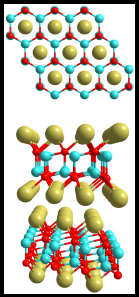The exemplary compound is β-LixZrNCl, for which optimal stoichiometry is identified as occurring at xopt = 0.08 at which point the full superconducting volume is reached and TC (= 15.1 K) is maximized (see [19, 20] of Ref. ). Underdoping occurs for x < 0.08, as identified by diminished superconducting volumes that vanish for x < 0.05 , indicating x0 = 0.05. Muon-spin-depolarization rates σμ(T → 0) for LixZrNCl are linear in (x − x0) with x0 = 0.05 obtained by extrapolation (see  in Ref. ; also, plasma frequency data show that ωp2 extrapolates to zero for x ≈ x0 (see  in Ref. . Signature characteristics of overdoping occur for x > xopt in that TC is a decreasing function of x, falling off precipitously from 15.1 K for x between 0.08 and 0.2 and reaching a minimum of about 11.5 K for 0.2 < x < 0.4 (see [19, 47] in Ref. ). This negates the notion of the superfluid density being a dominant factor in determining TC in the overdoped regime, as suggested elsewhere . Continuous incorporation of Li and charge doping have been concluded from an x dependence in certain Raman modes, particularly for in-plane vibrations of the [ZrNCl]2 block (mode denoted “A” in (see  of Ref. ). Within this x variation, the underdoping and overdoping regimes appear to be reflected in the x dependence of the lattice parameters (see  of Ref. ). The lattice parameter c is particularly sensitive to the intercalant thickness and dictated to a significant extent by the Li–Cl bond length that determines ζ, since d is given by c/3 while the [ZrNCl]2 thickness d2 tends to show little variation with intercalation. The variation of c vs. x is strongest at low x and crosses over to a weaker dependence for x greater than about 0.1, which is rather close to the optimal doping point xopt. This behavior suggests that participating charges are introduced into the interaction layers for x ≤ xopt with the equilibrium charge structure of the Li–Cl interaction layers fully formed at x = xopt. For x > xopt, the excess charges are transferred to the [ZrNCl]2 reservoir, yielding weak variation of intercalant thickness with the further increases in x. This non-participating charge fraction, being more localized in the [ZrN]2 structures and minimally affecting the Cl sites, is assumed benign with respect to the superconducting pairing interaction. A similar change in slope is revealed in the careful measurements of ωp2 vs. x, which nearly coincide with results from first principles calculations of band structure . In addition, the damping τ−1 is reported to be greater for x > xopt containing non-participating charge. Also reported in (see  in Ref. ) is the compound Zn0.04ZrNCl, with TC = 15 K, which is possibly a divalent doping analogue of Li0.08ZrNCl. The corresponding co-intercalated compound, Li0.13(DMF)yZrNCl (where DMF is (N, N)-dimethyl-formamide, C3H7NO), with TCmeas = 13.7 K (see  in Ref. ), was also found to be optimal .  As in the case of the related TiNCl compounds, ζ = (dd2)/2  and σ = γ |v[xopt – x0]|.  The γ factor is similarly obtained as well; a factor of (1/2) is given by rule (1b), and an additional two factors of (1/2) arises from the pairwise sharing between the Cl layer and the double-TiN-layered structure and then to the two TiN layers, such that γ = (1/2)(1/2)(1/2) = 1/8.  Thus :

• Type I reservoir: ZrNCl
• Type II reservoir: Lix(S)y
• note hexagonal structure
• σ = (1/2) (1/2) (1/2) |v [xopt – x0]|; v =1 for Li
• η = 1; ν = 2
• Li0.08ZrNCl: x0 = 0.05; A = 11.3233 Å2; ζ = 1.5817 Å; TC0calc = 14.35 K
• Li0.13(DMF)yZrNCl: x0 = 0; A = 11.3233 Å2; ζ = 3.400 Å; TC0calc = 13.90 K
1. D. R. Harshman and A. T. Fiory, J. Supercond. Nov. Magn. 28, 2967 (2015).

Ax(S)yHfNCl (TC0meas = 20 – 24 K)Calculation of σ utilizes the same methodology as is applied in the case of Lix(S)yZrNCl.

• Type I reservoir: HfNCl
• Type II reservoir: Ax(S)y
• note hexagonal structure
• σ = (1/2) (1/2) (1/2) |v [xopt – x0]|; v = 1
• η = 1; ν = 2
• Na0.25HfNCl: x0 = 0.15; A = 11.1484 Å2; ζ = 1.6580 Å; TC0calc = 25.19 K
• Li0.2HfNCl: x0 = 0.15; A = 11.1195 Å2; ζ = 31.5950 Å; TC0calc = 20.31 K
• Li0.2(NH3)yHfNCl: x0 = 0; A = 11.1117 Å2; ζ = 2.7620 Å; TC0calc = 21.42 K
• Ca0.11(NH3)yHfNCl: x0 = 0; A = 11.1251 Å2; ζ = 2.7370 Å; TC0calc = 22.66 K
• Eu0.08(NH3)yHfNCl: x0 = 0; A = 11.1117 Å2; ζ = 2.6690 Å; TC0calc = 24.28 K
1. D. R. Harshman and A. T. Fiory, J. Supercond. Nov. Magn. 28, 2967 (2015).

κ–[BEDT-TTF]2Cu[N(CN)2]Br (Pnma, TC0meas = 11.4 K)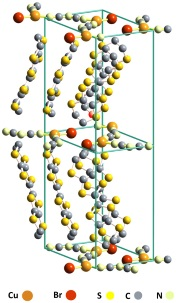For κ–[BEDT–TTF]2Cu[N(CN)2]Br , the hole conduction is in the ac-plane along the sulfur chains of the two BEDT-TTF molecules (each of which is bisected by a centrally located C–C  bond).  Equating the Cu+1[N(CN)2]–1Br–1 anion layer with Cu+2[O–2][O–2] (type II layer) shows a factor of 1/2 between the valences of the cuprate plane ions compared to those comprising the Cu[N(CN)2]Br anion molecule, leading to a base anion layer charge from rule (2c) of σ0/2, which must equal the positive charge available to the two BEDT-TTF (type I layer) molecules.  Dividing this charge between the two BEDT-TTF molecules comprising the dimer [rule (1a)], and further distributing the charge among the two halves of the BEDT-TTF molecule [rule (1a)], yields an additional factor of (1/2)(1/2) such that γ = (1/2)(1/2)(1/2) = 0.125.  Thus, one has :

• Type I reservoir: S-chains of the [BEDT-TTF]2 molecules
• Type II reservoir: Cu[N(CN)2]Br
• σ = (1/2) (1/4) σ0 = 0.125 σ0
• η = 1; ν = 2
• A = 54.4745 Å2; ζ = 2.4579 Å
• TC0calc = 11.61 K
1. D. R. Harshman, A. T. Fiory and J. D. Dow, J. Phys.: Condens. Matt. 23, 295701 (2011)23, 349501 (2011).

Cs3C60 (Pm3n, TC0meas = 38.3 K (A15, 0.93 GPa); Fm3m, 35.3 K (FCC, 0.73 GPa)) 

When viewed from a local perspective the surfaces of the C60 molecules of the 3D (A,B)3C60 macrostructure present an important adaptation of Coulombic pairing involving interactions between interfacial structures; the surface of the C60 molecule and the three alkali-metal cations located on an intervening virtual surface. Given the n-type character of these materials, one associates the superconducting condensate with the C60 molecules (type I reservoir), with the pairing mediated by holes on the cations (type II reservoir).  Both the A15 and FCC structures of Cs3C60 are Mott insulators at ambient-pressure, exhibiting antiferromagnetism with a Néel temperature of ~46 K and ~2 K, respectively [Takabayashi2009] [Ganin2008] [Ganin2010].  Under hydrostatic pressure, both phases become superconducting; optimization of the A15 and FCC phases occur at 0.93 and 0.73 GPa, respectively, with corresponding measured TC0 values of 38.36 K and 35.2 K [Takabayashi2009] [Ganin2010].

The C60 molecule has a diameter of 7.1 Å (radius R = 3.55 Å) [Hedberg1991], and comprises 12 regular pentagons, with a C–C bond length of 1.45 Å, and 20 hexagons, each with a weighted average C–C bond length of (1.45 + 1.40)/2 = 1.425 Å (the first term corresponding to the 6:5 bond and the second to the 6:6 (with equal weighting) [Pennington1996]), such that the total surface area of the C60 molecule at STP (assuming regular polygons) is A = 20(5.2757 Å2) + 12(3.6173 Å2) = 148.922 Å2.  For simplicity, A is assumed the same for all materials (and pressures [Kaur_arXiv_2007]) considered herein.  Since η = υ = 1 (where υ is the number of interacting type I layers/interfaces per f.u.), the γ factor is given by rule (1b) as, γ = 1/2, σ = (1/2) [x – x0], and Eq. (1) reduced to, TC0 = (72.28 K-Å) [x] ζ–1, with x = 3 for the stoichiometric (optimal) compounds [Haddon1993].  Note that all of this assumes an insulating (or near-insulating) end material.

For A15 Cs3C60, the three Cs cations are located at tetrahedral (T) sites facing the C60 hexagons [Ganin2008;suppl.] {check} (of average radius 1.425 Å) [Pennington1996].  In the fcc phase, on the other hand, the Cs cations are distributed between two interstitial locations; two Cs ions occupy the (T) sites, while the octahedral (O) site hosts the third [Ganin2010].  Notice that the Cs type II locations face unoccupied type I lattice positions along the radial, and can be related in 2D to defining ζ in, e.g., the β-form doped metal-hydride-nitrides [Harshman2015].  Designating  to be the (weighted) radial interaction distance for each of the N nearest neighbor Cs sites, the average interaction distance is then an average of the occupied nearest-neighbor sites [Note].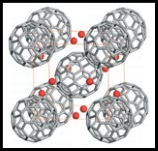A15 (BCC packing) structure Cs3C60 – Measurements conducted on a 77.7(6)% A15 enriched sample with stoichiometry Cs2.85(1)C60 (space group Pmn) and TC = 38.3 K (optimized at 0.93 GPa) show V0.93(TC) = 1533.6 Å3 and a0.93(TC) = 11.5320 Å [Takabayashi2009].  Determining ζ in the A15 compound is straight forward since Rietveld refinement of this structural phase indicates that the Cs cations occupy only the hexagon-coordinated  6d tetrahedral (T) sites; the 6c sites are left empty.  Given that the (T) position is located a distance 51/2a0/4 from the center of a C60 molecule, and faces the center of the hexagon of radius Rthe average interaction distance ζ = ζ(T) = 51/2a0.93/4 – (R2 – 1.4252)1/2 =  6.4463 – 3.2514 = 3.1952 Å.  From this, the above equation gives TC0calc. = 38.19 K in excellent agreement with experiment.  As no octahedral sites are occupied, the average over nearest-neighbor Cs cations is trivial.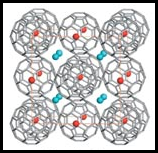The FCC packing structure Cs3C60 – At TC = 35.3 K and applied pressure of 0.73 GPa, the volume per unit cell for an 85.88(2)% fcc (Fmm space group) sample with stoichiometry Cs2.901(6)C60 is measured to be V0.73(TC) = 3038.4 Å3, corresponding to a lattice parameter of a0.73(TC) = 14.4838 Å [Ganin2010].  Unlike its A15 counterpart, the C60 molecules of the fcc phase exhibit merohedral disorder [Pennington1996] [Potocnik2014].  The type II surface comprises 8 Cs cations occupying the (T) sites (with four neighboring C60 molecules) and 6 in the octahedral (O) sites, also with six C60 neighbors; the number of Cs cations per C60 is then 8/4 + 6/6 = 3 [Note].  From Ref. [Potocnik2014], the (T) sites are located over the center of the C-hexagons a distance 31/2a0/4 from the C60 center, while the (O) sites lie above the midpoint of the 6:6 hexagon C-C bonds, a distance a0/2 from the C60 center.  Consequently, ζ(T) = 31/2a0.73/4 – (R2 – 1.4252)1/2 = 3.0203 Å, and ζ(O) = a0.73/2 – (R2 – 0.72)1/2 = 3.7616 Å.  Taking the weighted average then gives, ζ = [(8/14)ζ(T) + (6/14)ζ(O)] = 3.3380 Å, which from Eq. (3) gives, TC0calc. = 36.88 K.  While the agreement with experiment is reasonable, the difference is sufficiently large to suggest that pair breaking due to the merohedral disordering of the C60 molecules may be a factor.

Note: There’s a difference between the number of cation sites per unit cell (12 for fcc) and the number of cation sites that are the nearest neighbors to a given C60 (14 for fcc); one is interested in the latter, not the former when calculating ζ. Consider the C60 situated at coordinates (0,0,0) and its nearest neighboring Cs; the 8 tetrahedrally coordinated Cs sites (T) are located at (i,j,k)(a0/4) and the 6 octahedrally coordinated Cs sites (O) are located at (i,0,0)(a0/2), (0,j,0)(a0/2), and (0,0,k)(a0/2) (with i,j,k = +1 or –1).  This C60 is neighbored by 8 (T) Cs, shared by four C60s, so the number of (T) Cs per this C60 = 8/4 = 2.  And by 6 (O) Cs, shared by six C60 molecular anions, so the number of (O) Cs per this C60 is 6/6 = 1.  Thus the total number of Cs per C60 comes out correctly as 3.  The model for ζ is based on the type II shell of Cs surrounding a given C60.  The fractions of (T) and (O) Cs sites per C60 are inequivalent to the fractions of nearest-neighbor (T) and (O) sites, because of the model geometry and coordination sharing. In summary one has :

• Type I reservoir: C60
• Type II reservoir: A3
• η = 1; ν = 1
• σ = γ [3 –x0] = (1/2) [3 ] = 1.5 (assuming x0 = 0)
• [BCC]: A = 148.922 Å2; ζ = 3.1949 Å (assuming negligible pressure dependence in A); TC0meas = 38.36 K; TC0calc = 38.19 K (i.e., A15 phase)
• [FCC]: A = 148.922 Å2; ζ = 3.383 Å (assuming negligible pressure dependence in A); TC0meas = 35.2 K; T C0calc = 36.88 K
1. D. R. Harshman and A. T. Fiory, J. Phys.: Condens. Matter 29, 145602 (2017).
• Y. Takabayashi et al., Science 323, 1585 (2009).
• A. Y. Ganin et al., Nature Mater. 7, 367; suppl. (2008).
• A. Y. Ganin et al., Nature 466, 221; suppl. (2010).
• C. H. Pennington and V. A. Stenger, Rev. Mod. Phys. 68, 855 (1996).
• R. C. Haddon, Pure and Appl. Chem. 65, 11 (1993).
• A. Potocnik et al., Chem. Sci. 5, 3008 (2014).
• K. Hedberg et al., Science 254, 410 (1991).
• N. Kaur et al., arXiv (2007).

H3S (Im3m, TC0meas = 200.0 K (155 GPa))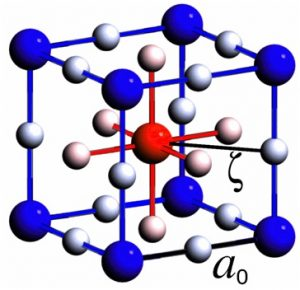The H3S system represents the first true 3D material for which the interfacial Coulombic interaction model has been applied.  It is also the first material in which the type I and type II charge reservoirs contain the same ionic species.

• Type I reservoir: half-sublattice
• Type II reservoir: half-sublattice
• η = 1; ν = 1
• σ = γ [x] = (1/2) [3.43 + 3.43] = 3.43
• A = 3a02 = 28.5017 Å2 (a0 = 3.0823 Å); ζ = a0/21/2 = 2.1795 Å; TC0meas = 200 K (155 GPa); TC0calc = 198.5 ± 3.0 K
1. D. R. Harshman and A. T. Fiory, J. Phys.: Condens. Matter 29, 445702 (2017).

Twisted Bilayer Graphene (gated) (TC0meas = 1.83(5) K at 0 GPa; 2.86(5) K at 1.33 GPa)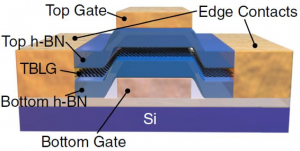The TBG system is an extended 2D lattice where, like the 3D compound H3S, the two reservoirs are essentially identical, containing both superconducting and mediating charges.

• Type I reservoir: first graphene sheet
• Type II reservoir: second graphene sheet
• η = 1; ν = 1
• θ = 1.05° (0 GPa); 1.27° (1.33 GPa)
• σ/A = γ |noptn0| = 1/2|noptn0|
• ζ = a0/21/2 = 3.5 Å; TC0meas = 1.83(5) K; TC0calc = 1.94(4) K at 0 GPa
• ζ = a0/21/2 = 3.42 Å; TC0meas = 2.86(5) K; TC0calc = 3.02(3) K at 1.33 GPa
1. D. R. Harshman and A. T. Fiory, J. Supercond. Nov. Magn. 33, 367 (2020).

Compressed LaH10 (TC0meas = 251(1) K at 169(4) GPa; 262(1) at 192(4) GPa)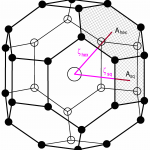The metal-hydrogen clathrate system is another 3D example where the mediating (type II) charges occupy the central metal ions and the superconducting (type I) charges occupy the surrounding hydrogen cage.

• Type I reservoir: H-cage
• Type II reservoir: La
• η = 1; ν = 1
• γ = 1/2
• σ = γ (10 + 3) = 0.5 (13) = 6.5
• 169(4) GPa: A = 50.29(27) Å2; ζ = 1.795(5)Å; TC0 = 249.8(1.3) K
• 192(4) GPa: A = 48.18(36) Å2; ζ =1.757(7) Å; TC0 = 260.7(2.0) K
1. D. R. Harshman and A. T. Fiory, J. Supercond. Nov. Magn. 33, 2945 (2020).

Compressed CSH7 (maximum TC0meas = 287.7±1.2 K at 267 GPa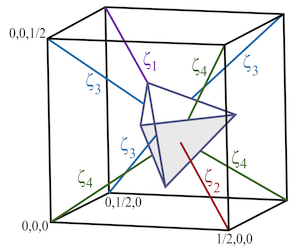For the 3D C-S-H system, mediating (type II) charges occupy the CH4 intercalates, and the superconducting (type I) charges occupy the surrounding H3S-like sub-lattice.

• Type I reservoir: H
• Type II reservoir: La
• η = 1; ν = 1
• γ = 1/2
• σ = γ (7 + 4 + 4) = 0.5 (115) = 7.5
• 267 GPa: A = 48.11(14) Å2; ζ = 1.737(4)Å; TC0 = 283.6±3.5 K
1. Dale R. Harshman and Anthony T. Fiory, Journal of Applied Physics 131, 015105 (2021); see also arXiv_v3 (2023).